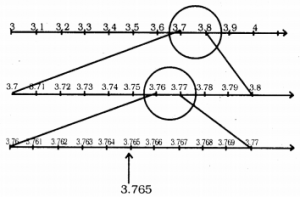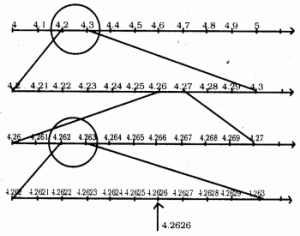# KSEEB Solutions for Class 9 Maths Chapter 1 Number Systems Ex 1.4

KSEEB Solutions for Class 9 Maths Chapter 1 Number Systems Ex 1.4 are part of KSEEB Solutions for Class 9 Maths. Here we have given Karnataka Board Class 9 Maths Chapter 1 Number Systems Exercise 1.4.

## Karnataka Board Class 9 Maths Chapter 1 Number Systems Ex 1.4

Question 11.
Visualize 3.765 on the number line, using successive magnification.Visualise $$4 . \overline{26}$$ on the number line, up to 4 decimal places.
Visulaisation of $$4 . \overline{26}$$ upto 4 decimal places on number line :